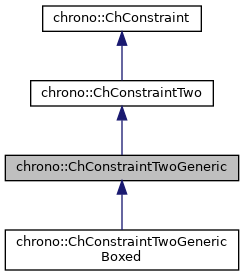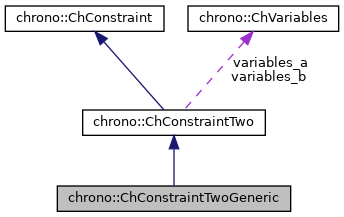chrono::ChConstraintTwoGeneric Class Reference

## Description

This class implements the functionality for a constraint between a COUPLE of TWO objects of type ChVariables(), with generic number of scalar variables each (ex.ChVariablesGeneric() or ChVariablesBody() ) and defines two constraint matrices, whose column number automatically matches the number of elements in variables vectors.

Before starting the solver one must provide the proper values in constraints (and update them if necessary), i.e. must set at least the c_i and b_i values, and jacobians.

#include <ChConstraintTwoGeneric.h>

Inheritance diagram for chrono::ChConstraintTwoGeneric:[legend]
Collaboration diagram for chrono::ChConstraintTwoGeneric:[legend]

## Public Member Functions

ChConstraintTwoGeneric ()
Default constructor.

ChConstraintTwoGeneric (ChVariables *mvariables_a, ChVariables *mvariables_b)
Construct and immediately set references to variables.

ChConstraintTwoGeneric (const ChConstraintTwoGeneric &other)
Copy constructor.

virtual ChConstraintTwoGenericClone () const override
"Virtual" copy constructor (covariant return type).

ChConstraintTwoGenericoperator= (const ChConstraintTwoGeneric &other)
Assignment operator: copy from other object.

virtual ChRowVectorRef Get_Cq_a () override
Access jacobian vector.

virtual ChRowVectorRef Get_Cq_b () override
Access jacobian vector.

virtual ChVectorRef Get_Eq_a () override
Access auxiliary vector (ex: used by iterative solvers).

virtual ChVectorRef Get_Eq_b () override
Access auxiliary vector (ex: used by iterative solvers).

virtual void SetVariables (ChVariables *mvariables_a, ChVariables *mvariables_b) override
Set references to the constrained objects, each of ChVariables type, automatically creating/resizing jacobians if needed.

virtual void Update_auxiliary () override
This function updates the following auxiliary data: More...

virtual double Compute_Cq_q () override
This function must computes the product between the row-jacobian of this constraint '[Cq_i]' and the vector of variables, 'v'. More...

virtual void Increment_q (const double deltal) override
This function must increment the vector of variables 'v' with the quantity [invM]*[Cq_i]'deltal,that is v+=[invM][Cq_i]'*deltal or better: v+=[Eq_i]*deltal This is used for some iterative solvers.

virtual void MultiplyAndAdd (double &result, const ChVectorDynamic< double > &vect) const override
Computes the product of the corresponding block in the system matrix by 'vect', and add to 'result'. More...

virtual void MultiplyTandAdd (ChVectorDynamic< double > &result, double l) override
Computes the product of the corresponding transposed blocks in the system matrix (ie. More...

virtual void Build_Cq (ChSparseMatrix &storage, int insrow) override
Puts the two jacobian parts into the 'insrow' row of a sparse matrix, where both portions of the jacobian are shifted in order to match the offset of the corresponding ChVariable.

virtual void Build_CqT (ChSparseMatrix &storage, int inscol) override
Same as Build_Cq, but puts the transposed jacobian row as a column.

virtual void ArchiveOUT (ChArchiveOut &marchive) override
Method to allow serialization of transient data to archives.

virtual void ArchiveIN (ChArchiveIn &marchive) override
Method to allow de-serialization of transient data from archives.Public Member Functions inherited from chrono::ChConstraintTwo
ChConstraintTwo ()
Default constructor.

ChConstraintTwo (const ChConstraintTwo &other)
Copy constructor.

ChConstraintTwooperator= (const ChConstraintTwo &other)
Assignment operator: copy from other object.

ChVariablesGetVariables_a ()
Access the first variable object.

ChVariablesGetVariables_b ()
Access the second variable object.Public Member Functions inherited from chrono::ChConstraint
ChConstraint ()
Default constructor.

ChConstraint (const ChConstraint &other)
Copy constructor.

ChConstraintoperator= (const ChConstraint &other)
Assignment operator: copy from other object.

bool operator== (const ChConstraint &other) const
Comparison (compares only flags, not the jacobians etc.)

virtual bool IsValid () const
Tells if the constraint data is currently valid.

virtual void SetValid (bool mon)
Use this function to set the valid state (child class Children classes must use this function depending on the result of their implementations of RestoreReference();.

virtual bool IsDisabled () const
Tells if the constraint is currently turned on or off by the user.

virtual void SetDisabled (bool mon)
User can use this to enable/disable the constraint as desired.

virtual bool IsRedundant () const
Tells if the constraint is redundant or singular.

virtual void SetRedundant (bool mon)
Solvers may use the following to mark a constraint as redundant.

virtual bool IsBroken () const
Tells if the constraint is broken, for excess of pulling/pushing.

virtual void SetBroken (bool mon)
3rd party software can set the 'broken' status via this method (by default, constraints never break);

virtual bool IsUnilateral () const
Tells if the constraint is unilateral (typical complementarity constraint).

virtual bool IsLinear () const
Tells if the constraint is linear (if non linear, returns false).

eChConstraintMode GetMode () const
Gets the mode of the constraint: free / lock / complementary A typical constraint has 'lock = true' by default.

void SetMode (eChConstraintMode mmode)
Sets the mode of the constraint: free / lock / complementary.

virtual bool IsActive () const
Tells if the constraint is currently active, in general, that is tells if it must be included into the system solver or not. More...

virtual void SetActive (bool isactive)
Set the status of the constraint to active.

virtual double Compute_c_i ()
Compute the residual of the constraint using the LINEAR expression. More...

double Get_c_i () const
Return the residual 'c_i' of this constraint. // CURRENTLY NOT USED.

void Set_b_i (const double mb)
Sets the known term b_i in [Cq_i]*q + b_i = 0, where: c_i = [Cq_i]*q + b_i = 0.

double Get_b_i () const
Return the known term b_i in [Cq_i]*q + b_i = 0, where: c_i= [Cq_i]*q + b_i = 0.

void Set_cfm_i (const double mcfm)
Sets the constraint force mixing term (default=0). More...

double Get_cfm_i () const
Returns the constraint force mixing term.

virtual void Set_l_i (double ml_i)
Sets the 'l_i' value (constraint reaction, see 'l' vector)

virtual double Get_l_i () const
Return the 'l_i' value (constraint reaction, see 'l' vector)

double Get_g_i () const
Return the 'g_i' product , that is [Cq_i]*[invM_i]*[Cq_i]' (+cfm)

void Set_g_i (double m_g_i)
Usually you should not use the Set_g_i function, because g_i should be automatically computed during the Update_auxiliary() .

virtual void Project ()
For iterative solvers: project the value of a possible 'l_i' value of constraint reaction onto admissible orthant/set. More...

virtual double Violation (double mc_i)
Given the residual of the constraint computed as the linear map mc_i = [Cq]*q + b_i + cfm*l_i , returns the violation of the constraint, considering inequalities, etc. More...

void SetOffset (int moff)
Set offset in global q vector (set automatically by ChSystemDescriptor)

int GetOffset () const
Get offset in global q vector.

## Protected Attributes

ChRowVectorDynamic< double > Cq_a
The [Cq_a] jacobian of the constraint.

ChRowVectorDynamic< double > Cq_b
The [Cq_b] jacobian of the constraint.

ChVectorDynamic< double > Eq_a
The [Eq_a] product [Eq_a]=[invM_a]*[Cq_a]'.

ChVectorDynamic< double > Eq_b
The [Eq_a] product [Eq_b]=[invM_b]*[Cq_b]'.Protected Attributes inherited from chrono::ChConstraintTwo
ChVariablesvariables_a
The first constrained object.

ChVariablesvariables_b
The second constrained object.Protected Attributes inherited from chrono::ChConstraint
double c_i
The 'c_i' residual of the constraint (if satisfied, c must be 0)

double l_i
The 'l_i' lagrangian multiplier (reaction)

double b_i
The 'b_i' right term in [Cq_i]*q+b_i=0 , note: c_i= [Cq_i]*q + b_i.

double cfm_i
The constraint force mixing, if needed (usually is zero) to add some numerical 'compliance' in the constraint, that is the equation becomes: c_i= [Cq_i]*q + b_i + cfm*l_i =0; Example, it could be cfm = [k * h^2](^-1) where k is stiffness.

eChConstraintMode mode
mode of the constraint: free / lock / complementar

double g_i
'g_i' product [Cq_i]*[invM_i]*[Cq_i]' (+cfm)

int offset
offset in global "l" state vector (needed by some solvers)

## ◆ Compute_Cq_q()

 double chrono::ChConstraintTwoGeneric::Compute_Cq_q ( )
overridevirtual

This function must computes the product between the row-jacobian of this constraint '[Cq_i]' and the vector of variables, 'v'.

that is CV=[Cq_i]*v This is used for some iterative solvers.

Implements chrono::ChConstraint.

 void chrono::ChConstraintTwoGeneric::MultiplyAndAdd ( double & result, const ChVectorDynamic< double > & vect ) const
overridevirtual

Computes the product of the corresponding block in the system matrix by 'vect', and add to 'result'.

NOTE: the 'vect' vector must already have the size of the total variables&constraints in the system; the procedure will use the ChVariable offsets (that must be already updated) to know the indexes in result and vect;

Implements chrono::ChConstraint.

 void chrono::ChConstraintTwoGeneric::MultiplyTandAdd ( ChVectorDynamic< double > & result, double l )
overridevirtual

Computes the product of the corresponding transposed blocks in the system matrix (ie.

the TRANSPOSED jacobian matrix C_q') by 'l', and add to 'result'. NOTE: the 'result' vector must already have the size of the total variables&constraints in the system; the procedure will use the ChVariable offsets (that must be already updated) to know the indexes in result and vect;

Implements chrono::ChConstraint.

## ◆ Update_auxiliary()

 void chrono::ChConstraintTwoGeneric::Update_auxiliary ( )
overridevirtual

This function updates the following auxiliary data:

• the Eq_a and Eq_b matrices
• the g_i product This is often called by solvers at the beginning of the solution process. Most often, inherited classes won't need to override this.

Reimplemented from chrono::ChConstraint.

The documentation for this class was generated from the following files:
• /builds/uwsbel/chrono/src/chrono/solver/ChConstraintTwoGeneric.h
• /builds/uwsbel/chrono/src/chrono/solver/ChConstraintTwoGeneric.cpp